International
Tables for
Crystallography
Volume C
Mathematical, physical and chemical tables
Edited by E. Prince

International Tables for Crystallography (2006). Vol. C, ch. 5.2, p. 497

Table 5.2.7.1

W. Parrish,a A. J. C. Wilsonb and J. I. Langfordc

aIBM Almaden Research Center, San Jose, CA, USA,bSt John's College, Cambridge CB2 1TP, England, and cSchool of Physics & Astronomy, University of Birmingham, Birmingham B15 2TT, England

 Table 5.2.7.1| top | pdf | Centroid displacement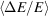and variance W of certain aberrations of an energy-dispersive diffractometer [mainly from Wilson (1973), where more detailed results are given for the aberrations marked with an asterisk]
 The Soller slits are taken to be in the original orientation (Soller, 1924). For the notation, see the footnote.
 AberrationW Specimen displacement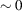Included in equatorial divergence Specimen transparency*? Equatorial divergence*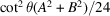for narrow Soller slits Axial divergence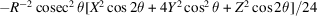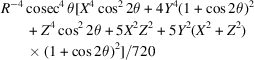Refraction* Probably negligible at the present stage of technique Response variations Centroid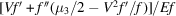? Peak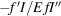? Interaction of Lorentz etc. factors and geometrical aberrations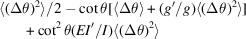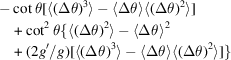Notation: A and B are the angular apertures (possibly equal) of the two sets of Soller slits; E is the energy of the detected photon; f (E) is the variation of a response (energy of the continuous radiation, absorption in the specimen etc.) with E; g(θ) is an angle-dependent response (Lorentz factor etc.); I(EE1) dE is the counting rate recorded at E when the energy of the incident photons is actually E1; R is the diffractometer radius; V is the variance and μ3 is the third central moment of the energy-resolution function I; 2X, 2Y, 2Z are the effective dimensions (possibly equal) of the source, specimen, and detector; the primes indicate differentiation; the averages <(Δθ)2> etc. are over the range of Bragg angles permitted by the slits etc.